# C++ Programs to Pass Array In Function

In this tutorial you will learn about the C++ Programs to Pass Array In Function and its application with practical example.

## C++ Programs to Array In Function

In this tutorial, we will learn to create a C++ program that will Pass Array In Function using C++ programming.

## Prerequisites

Before starting with this tutorial we assume that you are best aware of the following C++ programming topics:

• Operators in C++ Programming.
• Basic Input and Output function in C++ Programming.
• Basic C++ programming.
• For loop in C++ Programming.
• Functions in C++ Programming.

## Program to pass Array In Function:-

As we all know array is a collection of similar data type elements. we can perform many different operations on arrays in c++ programming. In today’s program, we will reverse a given array using a user-defined function. Bypassing that array to a function.

## Program Code:-

Pass Array In Function To Reverse Array Element Using Function

## Output:-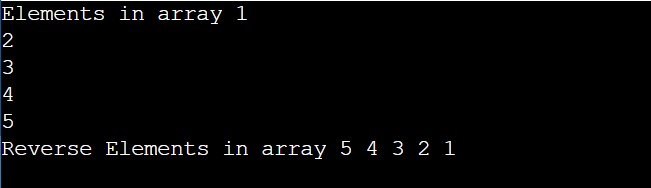In the above program, we have first initialized the required variable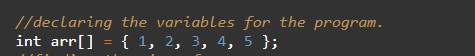• arr = it will hold the elements in an array.
• n = it will hold the number of elements in an array.
• i = it will hold the integer value to control the array.

Printing elements of the array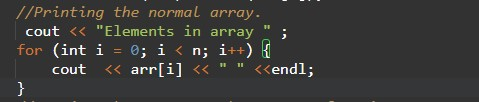Calling of user-defined function in the program.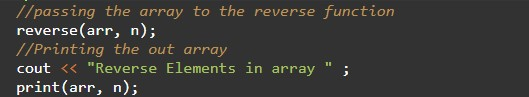Program Code for reverse function & Print function.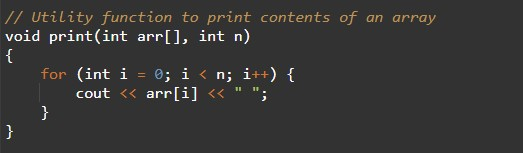In this tutorial we have learn about the C++ Programs to Pass Array In Function and its application with practical example. I hope you will like this tutorial.